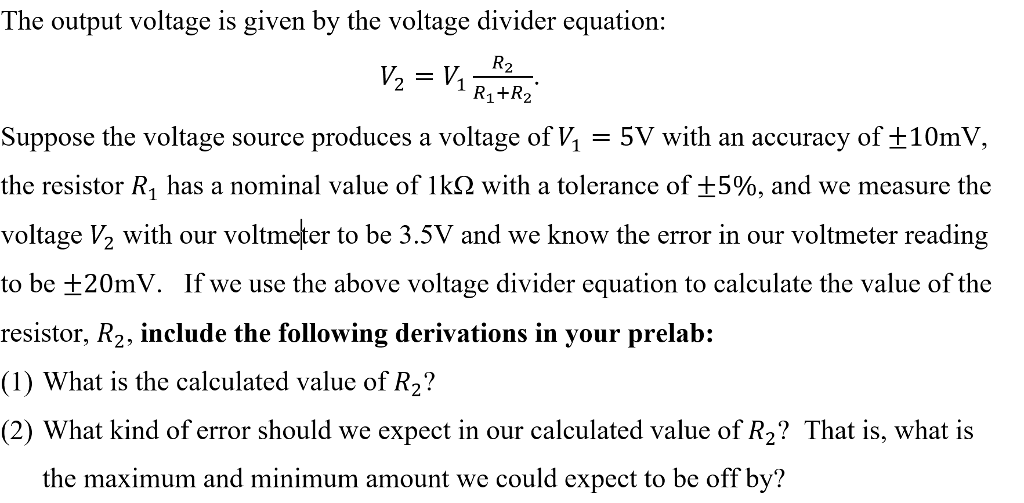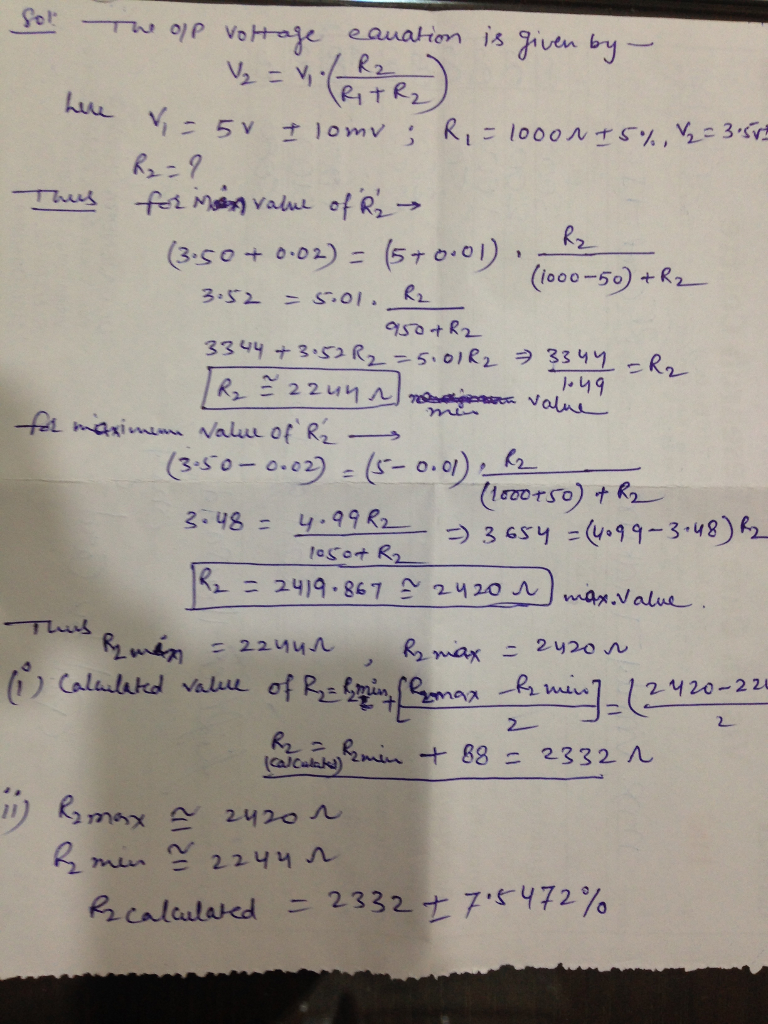# Homework Solution: The output voltage is given by the voltage divider equation: V_2 = V_1 R_2/R_1 + R_2. Suppose the voltage source produces a volta…The output voltage is given by the voltage divider equation: V_2 = V_1 R_2/R_1 + R_2. Suppose the voltage source produces a voltage of V_1 = 5V with an accuracy of plusminus 10mV, the resistor R_1 has a nominal value of 1k ohm with a tolerance of plusminus 5%, and we measure the voltage V_2 with our voltmeter to be 3.5V and we know the error in our voltmeter reading to be plusminus 20mV. If we use the above voltage divider equation to calculate the value of the resistor, R_2, include the following derivations in your prelab: What is the calculated value of R_2? What kind of error should we expect in our calculated value of R? That is, what is the maximum and minimum amount we could expect to be off by?